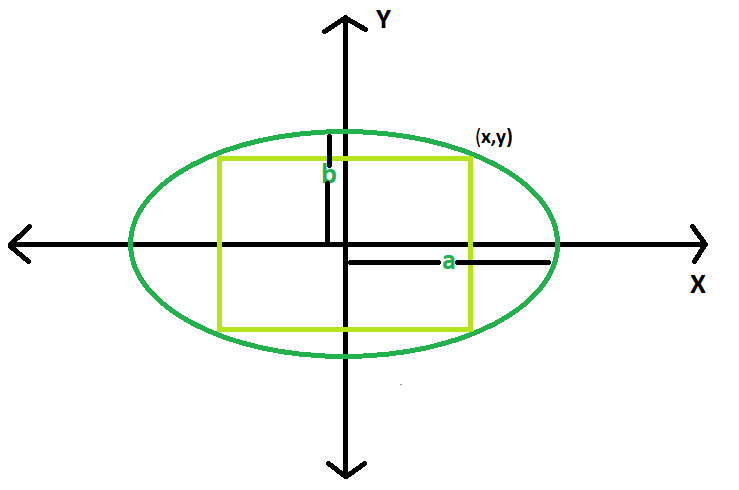# Area of Largest rectangle that can be inscribed in an Ellipse

Given an ellipse, with major axis length 2a & 2b. The task is to find the area of the largest rectangle that can be inscribed in it.

Examples:

```Input: a = 4, b = 3
Output: 24

Input: a = 10, b = 8
Output: 160
```

## Recommended: Please try your approach on {IDE} first, before moving on to the solution.Approach:
Let the upper right corner of the rectangle has co-ordinates (x, y),
Then the area of rectangle, A = 4*x*y.

Now,

Equation of ellipse, (x2/a2) + (y2/b2) = 1
Thinking of the area as a function of x, we have
dA/dx = 4xdy/dx + 4y

Differentiating equation of ellipse with respect to x, we have
2x/a2 + (2y/b2)dy/dx = 0,

so,
dy/dx = -b2x/a2y,
and

Setting this to 0 and simplifying, we have y2 = b2x2/a2.

From equation of ellipse we know that,
y2=b2 – b2x2/a2

Thus, y2=b2 – y2, 2y2=b2, and y2b2 = 1/2.

Clearly, then, x2a2 = 1/2 as well, and the area is maximized when
x= a/√2 and y=b/√2

So the maximum area Area, Amax = 2ab

Below is the implementation of the above approach:

## C++

 `// C++ Program to find the biggest rectangle ` `// which can be inscribed within the ellipse ` `#include ` `using` `namespace` `std; ` ` `  `// Function to find the area ` `// of the rectangle ` `float` `rectanglearea(``float` `a, ``float` `b) ` `{ ` ` `  `    ``// a and b cannot be negative ` `    ``if` `(a < 0 || b < 0) ` `        ``return` `-1; ` ` `  `    ``// area of the rectangle ` `    ``return` `2 * a * b; ` `} ` ` `  `// Driver code ` `int` `main() ` `{ ` `    ``float` `a = 10, b = 8; ` `    ``cout << rectanglearea(a, b) << endl; ` `    ``return` `0; ` `} `

## Java

 `// Java Program to find the biggest rectangle ` `// which can be inscribed within the ellipse ` ` `  `import` `java.util.*; ` `import` `java.lang.*; ` `import` `java.io.*; ` ` `  `class` `GFG{  ` `// Function to find the area ` `// of the rectangle ` `static` `float` `rectanglearea(``float` `a, ``float` `b) ` `{ ` `  `  `    ``// a and b cannot be negative ` `    ``if` `(a < ``0` `|| b < ``0``) ` `        ``return` `-``1``; ` `  `  `    ``// area of the rectangle ` `    ``return` `2` `* a * b; ` `} ` `  `  `// Driver code ` `public` `static` `void` `main(String args[]) ` `{ ` `    ``float` `a = ``10``, b = ``8``; ` `    ``System.out.println(rectanglearea(a, b)); ` `} ` `} `

## Python 3

 `# Python 3 Program to find the biggest rectangle  ` `# which can be inscribed within the ellipse  ` ` `  `#  Function to find the area  ` `# of the rectangle  ` `def` `rectanglearea(a, b) : ` ` `  `    ``# a and b cannot be negative  ` `    ``if` `a < ``0` `or` `b < ``0` `: ` `        ``return` `-``1` ` `  `    ``# area of the rectangle ` `    ``return` `2` `*` `a ``*` `b ` `  `  ` `  `# Driver code      ` `if` `__name__ ``=``=` `"__main__"` `: ` ` `  `    ``a, b ``=` `10``, ``8` `    ``print``(rectanglearea(a, b)) ` ` `  ` `  `# This code is contributed by ANKITRAI1 `

## C#

 `// C# Program to find the ` `// biggest rectangle which  ` `// can be inscribed within ` `// the ellipse ` `using` `System; ` ` `  `class` `GFG ` `{  ` `// Function to find the area ` `// of the rectangle ` `static` `float` `rectanglearea(``float` `a,  ` `                           ``float` `b) ` `{ ` ` `  `    ``// a and b cannot be negative ` `    ``if` `(a < 0 || b < 0) ` `        ``return` `-1; ` ` `  `    ``// area of the rectangle ` `    ``return` `2 * a * b; ` `} ` ` `  `// Driver code ` `public` `static` `void` `Main() ` `{ ` `    ``float` `a = 10, b = 8; ` `    ``Console.WriteLine(rectanglearea(a, b)); ` `} ` `} ` ` `  `// This code is contributed  ` `// by inder_verma `

## PHP

 ` `

Output:

```160
```

Attention reader! Don’t stop learning now. Get hold of all the important DSA concepts with the DSA Self Paced Course at a student-friendly price and become industry ready.

My Personal Notes arrow_drop_upCheck out this Author's contributed articles.

If you like GeeksforGeeks and would like to contribute, you can also write an article using contribute.geeksforgeeks.org or mail your article to contribute@geeksforgeeks.org. See your article appearing on the GeeksforGeeks main page and help other Geeks.

Please Improve this article if you find anything incorrect by clicking on the "Improve Article" button below.